# sklearn.metrics.silhouette_score¶

sklearn.metrics.silhouette_score(X, labels, *, metric='euclidean', sample_size=None, random_state=None, **kwds)[source]

Compute the mean Silhouette Coefficient of all samples.

The Silhouette Coefficient is calculated using the mean intra-cluster distance (a) and the mean nearest-cluster distance (b) for each sample. The Silhouette Coefficient for a sample is (b - a) / max(a, b). To clarify, b is the distance between a sample and the nearest cluster that the sample is not a part of. Note that Silhouette Coefficient is only defined if number of labels is 2 <= n_labels <= n_samples - 1.

This function returns the mean Silhouette Coefficient over all samples. To obtain the values for each sample, use silhouette_samples.

The best value is 1 and the worst value is -1. Values near 0 indicate overlapping clusters. Negative values generally indicate that a sample has been assigned to the wrong cluster, as a different cluster is more similar.

Read more in the User Guide.

Parameters:
X{array-like, sparse matrix} of shape (n_samples_a, n_samples_a) if metric == “precomputed” or (n_samples_a, n_features) otherwise

An array of pairwise distances between samples, or a feature array.

labelsarray-like of shape (n_samples,)

Predicted labels for each sample.

metricstr or callable, default=’euclidean’

The metric to use when calculating distance between instances in a feature array. If metric is a string, it must be one of the options allowed by metrics.pairwise.pairwise_distances. If X is the distance array itself, use metric="precomputed".

sample_sizeint, default=None

The size of the sample to use when computing the Silhouette Coefficient on a random subset of the data. If sample_size is None, no sampling is used.

random_stateint, RandomState instance or None, default=None

Determines random number generation for selecting a subset of samples. Used when sample_size is not None. Pass an int for reproducible results across multiple function calls. See Glossary.

**kwdsoptional keyword parameters

Any further parameters are passed directly to the distance function. If using a scipy.spatial.distance metric, the parameters are still metric dependent. See the scipy docs for usage examples.

Returns:
silhouettefloat

Mean Silhouette Coefficient for all samples.

References

## Examples using sklearn.metrics.silhouette_score¶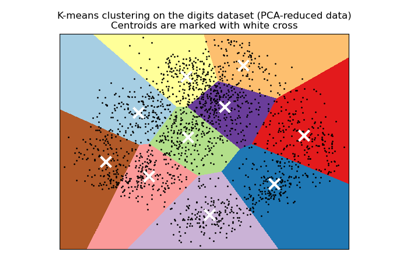A demo of K-Means clustering on the handwritten digits data

A demo of K-Means clustering on the handwritten digits data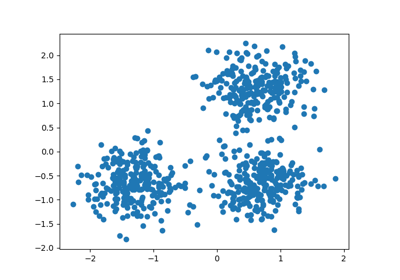Demo of DBSCAN clustering algorithm

Demo of DBSCAN clustering algorithm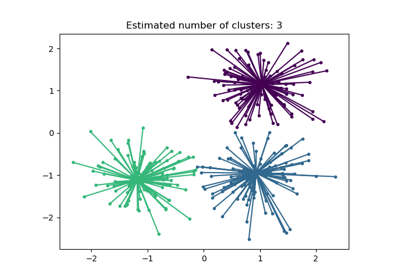Demo of affinity propagation clustering algorithm

Demo of affinity propagation clustering algorithm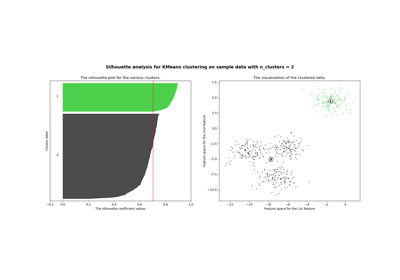Selecting the number of clusters with silhouette analysis on KMeans clustering

Selecting the number of clusters with silhouette analysis on KMeans clustering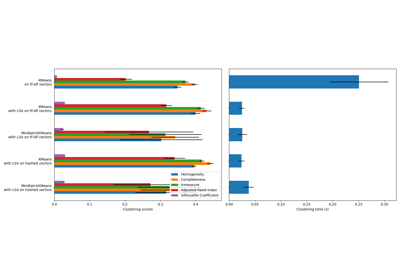Clustering text documents using k-means

Clustering text documents using k-means